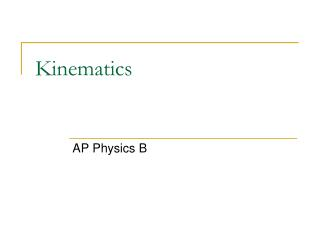DownloadDownload PresentationKinematics

# Kinematics

Télécharger la présentation## Kinematics

- - - - - - - - - - - - - - - - - - - - - - - - - - - E N D - - - - - - - - - - - - - - - - - - - - - - - - - - -
##### Presentation Transcript

1. Kinematics AP Physics B

2. Objective #1 • Objective: Introduction to kinematics • Before: Introduction to kinematics • During: Discuss kinematics • After: Review kinematics

3. Defining the important variables Kinematics is a way of describing the motion of objects without describing the causes. You can describe an object’s motion: In words Mathematically Pictorially Graphically No matter HOW we describe the motion, there are several KEY VARIABLES that we use.

4. The 3 Kinematic equations There are 3 major kinematic equations than can be used to describe the motion in DETAIL. All are used when the acceleration is CONSTANT.

5. Kinematic #1

6. Kinematic #1 Example:A boat moves slowly out of a marina (so as to not leave a wake) with a speed of 1.50 m/s. As soon as it passes the breakwater, leaving the marina, it throttles up and accelerates at 2.40 m/s/s. a) How fast is the boat moving after accelerating for 5 seconds? 13.5 m/s

7. Kinematic #2 b) How far did the boat travel during that time? 37.5 m

8. Does all this make sense? 13.5 m/s 1.5 m/s Total displacement = 7.50 + 30 = 37.5 m = Total AREA under the line.

9. Interesting to Note A = HB Most of the time, xo=0, but if it is not don’t forget to ADD in the initial position of the object. A=1/2HB

10. Kinematic #3 Example:You are driving through town at 12 m/s when suddenly a ball rolls out in front of your car. You apply the brakes and begin decelerating at 3.5 m/s/s. How far do you travel before coming to a complete stop? 20.57 m

11. Objective #2 • Objective: Understand motion in one dimension • Before: Introduction to motion in one dimension • During: Discuss motion in one dimension • After: Review motion in one dimension

12. Kinematics for the VERTICAL Direction All 3 kinematics can be used to analyze one dimensional motion in either the X direction OR the y direction.

13. “g” or ag – The Acceleration due to gravity The acceleration due to gravity is a special constant that exists in a VACUUM, meaning without air resistance. If an object is in FREE FALL, gravity will CHANGE an objects velocity by 9.8 m/s every second. • The acceleration due to gravity: • ALWAYS ACTS DOWNWARD • IS ALWAYS CONSTANT near the surface of Earth

14. Examples A stone is dropped at rest from the top of a cliff. It is observed to hit the ground 5.78 s later. How high is the cliff? Which variable is NOT given and NOT asked for? Final Velocity! -163.7 m H =163.7m

15. Examples A pitcher throws a fastball with a velocity of 43.5 m/s. It is determined that during the windup and delivery the ball covers a displacement of 2.5 meters. This is from the point behind the body when the ball is at rest to the point of release. Calculate the acceleration during his throwing motion. Which variable is NOT given and NOT asked for? TIME 378.5 m/s/s

16. Examples How long does it take a car at rest to cross a 35.0 m intersection after the light turns green, if the acceleration of the car is a constant 2.00 m/s/s? Which variable is NOT given and NOT asked for? Final Velocity 5.92 s

17. Examples A car accelerates from 12.5 m/s to 25 m/s in 6.0 seconds. What was the acceleration? Which variable is NOT given and NOT asked for? DISPLACEMENT 2.08 m/s/s